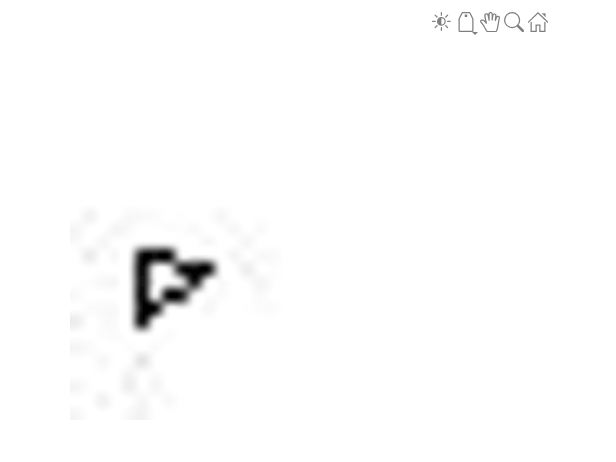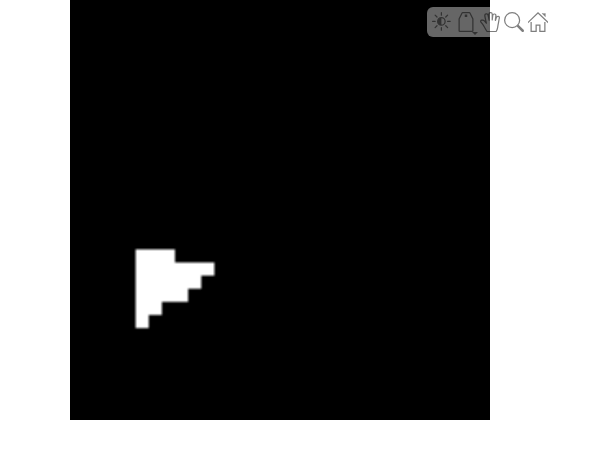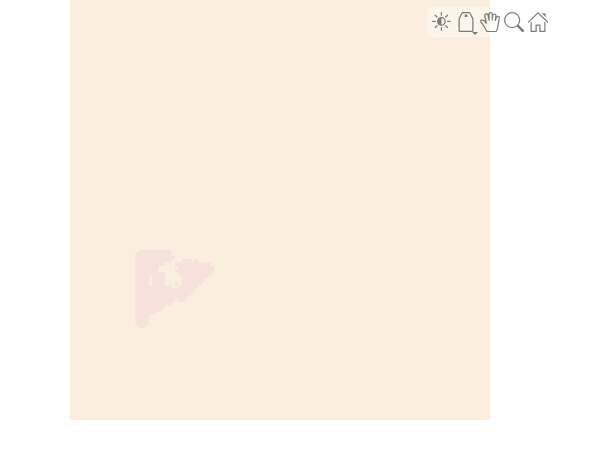# Calculate Segmentation Metrics in Block-Based Workflow

This example shows how to calculate the semantic segmentation confusion matrix for individual blocks in a `blockedImage` object, then calculate global and block segmentation metrics.

Load a pretrained network that performs binary segmentation of triangles against a background.

`load('triangleSegmentationNetwork');`

The `triangleImages` data set has 100 test images with ground truth labels. Define the location of the data set.

`dataSetDir = fullfile(toolboxdir('vision'),'visiondata','triangleImages');`

Define the location of the test images.

`testImagesDir = fullfile(dataSetDir,'testImages');`

Read three test images. Resize each image by a factor of four, convert it to data type `double`, then create a `blockedImage` object. A `blockedImage` supports block-based image processing workflows.

```numImages = 3; for idx = 1:numImages im = imread(fullfile(testImagesDir,['image_' '00' num2str(idx) '.jpg'])); im = imresize(im,4); testImages(idx) = blockedImage(im); end```

Display the first test image.

`bigimageshow(testImages(1))`Define the location of the ground truth labels.

`testLabelsDir = fullfile(dataSetDir,'testLabels');`

Define the class names and their associated label IDs.

```classNames = ["triangle","background"]; labelIDs = [255 0];```

Read in the ground truth labels for each test image. Create a `blockedImage` object from each ground truth label.

```for idx = 1:numImages gtLabel = imread(fullfile(testLabelsDir,['labeled_image_' '00' num2str(idx) '.png'])); gtLabel = imresize(gtLabel,4,'nearest'); groundTruthImages(idx) = blockedImage(gtLabel); end```

Display the first ground truth image.

`bigimageshow(groundTruthImages(1))`For each test image, use the `apply` function to process each block. The `apply` function performs the operations specified by the helper function `segmentAndCalculateBlockMetrics`, which is defined at the end of this example. The function performs semantic segmentation of each block and calculates the confusion matrix between the predicted and ground truth labels.

```blockSize = [32 32]; datasetConfMat = table; for idx = 1:numImages [segmentedImages(idx),blockConfMatOneImage] = apply(testImages(idx), ... @(block,labeledImageBlock) segmentAndCalculateBlockMetrics(block,labeledImageBlock,net,classNames,labelIDs), ... 'ExtraImages',groundTruthImages(idx),'PadPartialBlocks',true,'BlockSize',blockSize,'UseParallel',false); % Read all the block results of an image and update the image number blockConfMatOneImageDS = blockedImageDatastore(blockConfMatOneImage); blockConfMat = readall(blockConfMatOneImageDS); blockConfMat = struct2table([blockConfMat{:}]); blockConfMat.ImageNumber = idx.*ones(height(blockConfMat),1); datasetConfMat = [datasetConfMat;blockConfMat]; end```

Display the first segmented image.

`bigimageshow(segmentedImages(1))`Evaluate the data set metrics and block metrics for the segmentation.

`[metrics,blockMetrics] = evaluateSemanticSegmentation(datasetConfMat,classNames,'Metrics','all');`
```Evaluating semantic segmentation results ---------------------------------------- * Selected metrics: global accuracy, class accuracy, IoU, weighted IoU. * Processed 3 images. * Finalizing... Done. * Data set metrics: GlobalAccuracy MeanAccuracy MeanIoU WeightedIoU ______________ ____________ _______ ___________ 0.95428 0.82739 0.69927 0.92533 ```

Calculate the Jaccard score for all images.

`jaccardSimilarity = metrics.ImageMetrics.MeanIoU`
```jaccardSimilarity = 3×1 0.7664 0.7277 0.6538 ```

### Supporting Function

The `segmentAndCalculateBlockMetrics` function performs semantic segmentation of a single block then calculates the confusion matrix of the predicted and ground truth labels.

```function [outputLabeledImageBlock,blockConfMatPerBlock] = segmentAndCalculateBlockMetrics(block,labeledImageBlock,net,classNames,labelIDs) outputLabeledImageBlock = semanticseg(block.Data,net); % Convert the ground truth labels to categorical labeledImageBlock = categorical(labeledImageBlock,labelIDs,classNames); confusionMatrix = segmentationConfusionMatrix(outputLabeledImageBlock,labeledImageBlock); % blockConfMatPerBlock is a struct with confusion matrices, image number % and blockInfo. Use the struct with evaluateSemanticSegmentation to % calculate metrics and aggregate block-based results. blockConfMatPerBlock.ConfusionMatrix = confusionMatrix; blockConfMatPerBlock.ImageNumber = block.ImageNumber; blockInfo.Start = block.Start; blockInfo.End = block.End; blockConfMatPerBlock.BlockInfo = blockInfo; end```